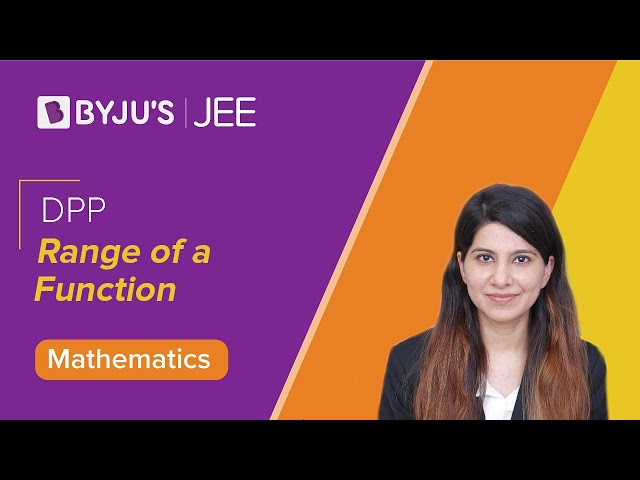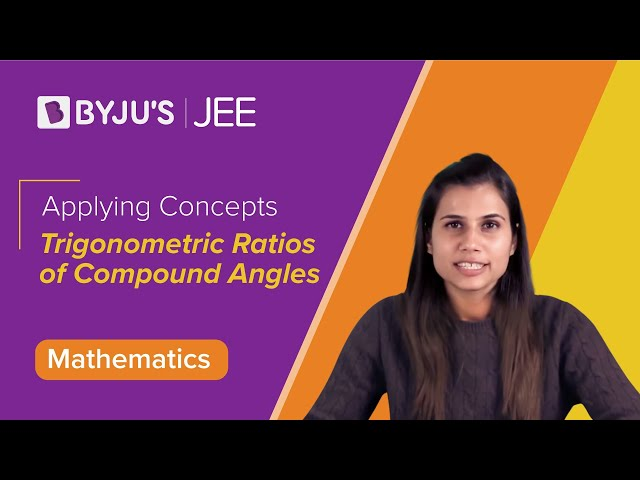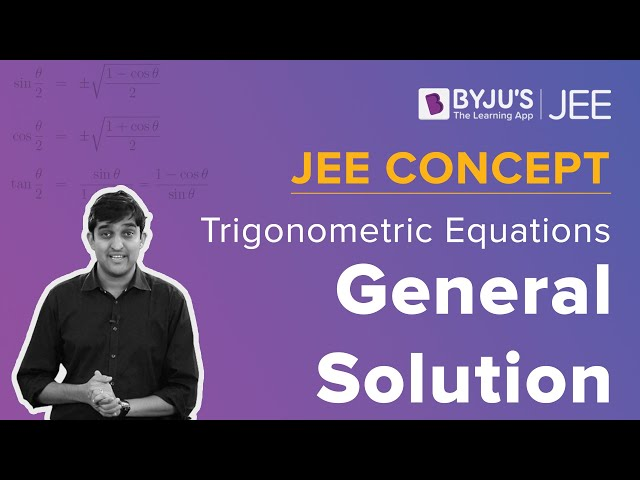Win up to 100% scholarship on Aakash BYJU'S JEE/NEET courses with ABNAT Win up to 100% scholarship on Aakash BYJU'S JEE/NEET courses with ABNAT

# JEE Main 2020 Maths Paper With Solutions Shift 1 Sept 5

JEE Main 2020 Maths paper September 5 Shift 1 solutions are available on this page. These step by step solutions are prepared by subject experts at BYJU’S. Practising these solutions will help students to improve their speed and accuracy. Students are recommended to revise and learn these so that they can score higher ranks for the JEE Main exam. The solutions are also available in PDF format for free.

## JEE Main 2020 Maths Paper With Solutions Shift 1 September 5

September 5 Shift 1 – Maths

1. If the volume of a parallelopiped, whose coterminuous edges are given by the vectors

$$\begin{array}{l}\vec{a}=\hat{i}+\hat{j}+n\hat{k}\end{array}$$
,
$$\begin{array}{l}\vec{b}=2\hat{i}+4\hat{j}-n\hat{k}\end{array}$$
, and
$$\begin{array}{l}\vec{c}=\hat{i}+n\hat{j}+3\hat{k}\end{array}$$
(n
$$\begin{array}{l}\geq\end{array}$$
0), is 158 cu. units, then:

a)

$$\begin{array}{l}\vec{a}.\vec{c}=17\end{array}$$

b)
$$\begin{array}{l}\vec{b}.\vec{c}=10\end{array}$$

c) n = 9
d) n = 7

$$\begin{array}{l}\begin{vmatrix} 1 & 1 &n \\ 2 & 4& -n\\ 1 & n& 3 \end{vmatrix}\end{array}$$
= 158

(12+n2)-(6+n)+n(2n-4) = 158

3n2-5n+6-158 = 0

3n2-5n-152 = 0

3n2-24n+19n-152 = 0

(3n+19)(n-8) = 0

⇒ n = 8

$$\begin{array}{l}\vec{b}.\vec{c}=10\end{array}$$

2. A survey shows that 73% of the persons working in an office like coffee, whereas 65% like tea. If x denotes the percentage of them, who like both coffee and tea, then x cannot be:

a) 63
b) 54
c) 38
d) 36

C → Coffee and T → Tea

n(C) = 73, n(T) = 65

n(coffee) = 73/100

n(tea) = 65/100

n(T∩C) = x/100

n(C∪T) = n(C)+n(T)-x

$$\begin{array}{l}\leq\end{array}$$
100

= 73+65-x

$$\begin{array}{l}\leq\end{array}$$
100

⇒ x

$$\begin{array}{l}\geq\end{array}$$
38

⇒ 73-x

$$\begin{array}{l}\geq\end{array}$$
0

⇒ x

$$\begin{array}{l}\leq\end{array}$$
73

⇒ 65-x

$$\begin{array}{l}\geq\end{array}$$
0

⇒ x

$$\begin{array}{l}\leq\end{array}$$
65

38

$$\begin{array}{l}\leq\end{array}$$
x
$$\begin{array}{l}\leq\end{array}$$
65

x = 36

x

$$\begin{array}{l}\leq\end{array}$$
min(n(C), n(T))
$$\begin{array}{l}\geq\end{array}$$
38
$$\begin{array}{l}\leq\end{array}$$
x
$$\begin{array}{l}\leq\end{array}$$
65

3. The mean and variance of 7 observations are 8 and 16, respectively. If five observations are 2,4,10,12,14, then the absolute difference of the remaining two observations is:

a) 1
b) 4
c) 3
d) 2

Var(x) =

$$\begin{array}{l}\sum \frac{x_{i}^{2}}{n}-(\bar{x})^{2}\end{array}$$

16 = (x12+ x22+ x42+ x52+ x62+ x72)/7 -64

80×7 = x12+ x22+ x32+…+ x72

Now, x62+ x72 = 560-(x12+…x52)

x62+x72 = 560-(4+16+100+144+196)

x62+x72 = 100 ..(i)

Now, (x1+x2+…+x7)/7 = 8

x6+x7 = 14

from (i) and (ii)

(x6+x7)2-2x6x7 = 100

2x6x7 = 96

⇒ x6x7 = 48 ..(iii)

Now, |x6-x7| = √((x6+x7)2-4x6x7)

= √(196-192)

= 2

4. If 210+29×31+28×32+…..+2×39+310 = S-211, then S is equal to:

a) 311
b) (311/2)+210
c) 2×311
d) 311-212

Let S’ = 210+29×31+ 28×32+—–+2×39+310 ..(i)

3×S’/2 = 29×31+28×32+…+310+311/2 ..(ii)

Equation (i) – (ii)

-S’/2 = 210-311/2

S’ = 311-211

Now S’= S-211

S = 311

5. If 32 sin2α-1,14 and 34-2 sin2α are the first three terms of an A.P. for some α , then the sixth term of this A.P. is:

a) 65
b) 81
c) 78
d) 66

28 = 32sin2α-1+34-2 sin2α

28 = 9sin2α/3 + 81/9sin2α

Let 9sin2α = t

28 = (t/3)+(81/t)

t2-84t+243 = 0

t2-81t-3t+243 = 0

t(t -81)-3(t-81) = 0

(t -81)(t-3) = 0

t = 81, 3

9sin2α = 92 or 3

sin2α = 1/2, 2 (rejected)

First term, a = 32sin2α-1

a = 1

Second term = 14

Common difference, d = 13

T6 = a+5d

T6 = 1+5×13 = 66

6. If the common tangent to the parabolas, y2 = 4x and x2 = 4y also touches the circle, x2+y2 = c2, then c is equal to:

a) 1/2
b) 1/4
c) 1/√2
d) 1/2√2

y = mx+1/m

x2 = 4(mx+1/m)

x2 -4mx-4/m = 0

D = 0

16m2+16/m = 0

16(m3+1)/m = 0

m = -1

⇒ y+x = -1

Now, |-1/√2| = |c|

c =

$$\begin{array}{l}\pm\end{array}$$
1/√2

7. If the minimum and the maximum values of the function f: [π/4, π/2] → R defined by f(θ) =

$$\begin{array}{l}\begin{vmatrix} -\sin ^{2}\theta & -1-\sin ^{2}\theta & 1\\ -\cos ^{2}\theta &-1- \cos ^{2}\theta & 1\\ 12 & 10 & -2 \end{vmatrix}\end{array}$$
are m and M respectively, then the ordered pair (m,M) is equal to :

a) (0,4)
b) (-4,0)
c) (-4,4)
d) (0,2√2)

f(θ) =

$$\begin{array}{l}\begin{vmatrix} -\sin ^{2}\theta & -1-\sin ^{2}\theta & 1\\ -\cos ^{2}\theta &-1- \cos ^{2}\theta & 1\\ 12 & 10 & -2 \end{vmatrix}\end{array}$$

C1 → C1-C2

C3 → C3+C2

=

$$\begin{array}{l}\begin{vmatrix} 1 & -1-\sin ^{2}\theta & -\sin ^{2}\theta \\ 1 &-1- \cos ^{2}\theta & -\cos ^{2}\theta \\ 2& 10 & 8 \end{vmatrix}\end{array}$$

C2 → C2-C3

=

$$\begin{array}{l}\begin{vmatrix} 1 & -1 & -\sin ^{2}\theta \\ 1 &-1 & -\cos ^{2}\theta \\ 2& 2 & 8 \end{vmatrix}\end{array}$$

= 1(2cos2 θ-8)+(8+2cos2 θ) -4sin2θ

f(θ) = 4cos 2θ

8. Let λ∈R. the system of linear equations

2x1-4x2+λx3 = 1

x1-6x2+x3 = 2

λx1-10x2+4x3 = 3

is inconsistent for:

a) exactly two values of λ
b) exactly one negative value of λ.
c) every value of λ.
d) exactly one positive value of λ.

D =

$$\begin{array}{l}\begin{vmatrix} 2 & -4 & \lambda \\ 1& -6& 1\\ \lambda &-10 & 4 \end{vmatrix}\end{array}$$

= 2(3λ+ 2)( λ-3)

D1 = -2(λ-3)

D2 = -2(λ+1)( λ-3)

D3 = –2(λ-3)

When λ = 3, then

D = D1 = D2 = D3 = 0

⇒ Infinite many solution

When λ = –2/3 then D1, D2, D3 none of them is zero so equations are inconsistent

λ = –2/3

9. If the point P on the curve, 4x2+5y2 = 20 is farthest from the point Q(0, -4), then PQ2 is equal to:

a) 48
b) 29
c) 21
d) 36

Given ellipse is (x2/5)+(y2/4) = 1

Let point P is (√5 cos θ, 2 sin θ)

(PQ)2 = 5cos2 θ+ 4(sin θ + 2)2

(PQ)2 = cos2θ+ 16 sin θ + 20

(PQ)2 = -sin2θ+ 16 sin θ + 21

= 85 -(sin θ – 8)2

Will be maximum when sin θ = 1

(PQ)2max = 85-49 = 36

10. The product of the roots of the equation 9x2-18|x|+5 = 0 is :

a) 25/81
b) 5/9
c) 5/27
d) 25/9

Let |x| = t

9t2-18t +5 = 0

9t2 -15t -3t+5 = 0

(3t -5)(3t-1) = 0

|x| = 5/3, 1/3

⇒ x = 5/3, -5/3, 1/3, -1/3

⇒ Product = 25/81

11. If y = y(x) is the solution of the differential equation ((5+ex)/(2+y))(dy/dx)+ex = 0 satisfying y(0) = 1, then a value of y(loge13) is:

a) 1
b) 0
c) 2
d) -1

((5+ex)/(2+y))(dy/dx) = -ex

∫dy/(2+y) = ∫-e-x/(ex+5) dx

ln (y+2) = -ln(ex+5) + C

(y+2) (ex+5) = C

Since y(0) = 1

⇒ C = 18

y+2 = 18/ex+5

at x = ln 13

y+2 = 18/(13+5) = 1

y = -1

12. If S is the sum of the first 10 terms of the series tan-1(1/3) + tan-1(1/7) + tan-1(1/13) + tan-1(1/21) +…, then tan(S) is equal to:

a) 5/11
b) 5/6
c) -6/5
d) 10/11

S =

$$\begin{array}{l}\tan ^{-1}\left ( \frac{1}{1+1\times 2} \right )+\tan ^{-1}\left ( \frac{1}{1+2\times 3} \right )+…\end{array}$$

Tr =

$$\begin{array}{l}\tan ^{-1}\left ( \frac{1}{1+r(r+1)} \right )\end{array}$$

Tr = tan-1(r+1)-tan-1r

T1 = tan-12-tan-11

T2 = tan-13-tan-12

T3 = tan-14-tan-13

T10 = tan-111-tan-110

S = (tan-12-tan-11) + (tan-1 3-tan-12) + (tan-14-tan-13) + ……… + (tan-1 11-tan-110)

S = tan-111-tan-11

= tan-1 [(11-1)/(1+11)]

⇒ tan S = 10/12

= 5/6

13. The value of

$$\begin{array}{l}\int_{\frac{-\pi }{2}}^{\frac{\pi }{2}}\frac{1}{1+e^{\sin x}}dx\end{array}$$
is:

a) π/2
b) π/4
c) π
d) 3π/2

I =

$$\begin{array}{l}\int_{\frac{-\pi }{2}}^{\frac{\pi }{2}}\frac{1}{1+e^{\sin x}}dx\end{array}$$

=

$$\begin{array}{l}\int_{\frac{-\pi }{2}}^{\frac{\pi }{2}}\frac{e^{\sin x}}{1+e^{\sin x}}dx\end{array}$$

⇒ 2I = π

I = π/2

14. If (a, b, c) is the image of the point (1,2,-3) in the line, (x+1)/2 = (y-3)/-2 = z/-1 then a+b+c is:

a) 2
b) 3
c) -1
d) 1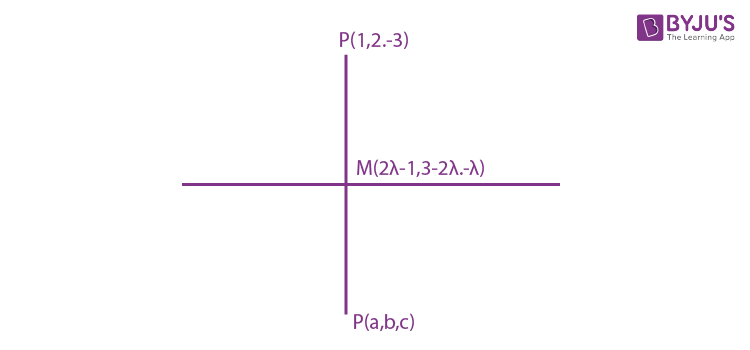Vector PM and (

$$\begin{array}{l}2\hat{i}-2\hat{j}-\hat{k}\end{array}$$
) are perpendicular.

⇒ (2λ-2)2+(1-2λ)(-2)+(3-λ) (-1) = 0

⇒ 4λ-4+4 λ-2+λ-3 = 0

⇒ 9λ = 9

⇒ λ = 1

⇒ M(1,1,-1)

Now, P’ = 2M-P

= 2(1,1,-1)-(1, 2, -3)

= (1, 0, 1)

a+b+c = 2

15. If the function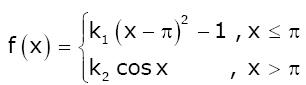is twice differentiable, then the ordered pair (k1, k2) is equal to:

a) (1,1)
b) (1,0)
c) (1/2, -1)
d) (1/2, 1)

f(x) is continuous and differentiable

f(π) = f(π) = f(π+)

-1 = -k2

⇒ k2 = 1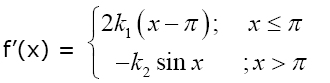f'(π) = f’(π+)

⇒ 0 = 0

So differentiable at x = 0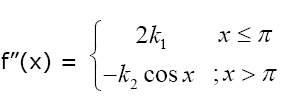f’’(π) = f’’(π+)

2k1 = k2

k1 = 1/2

16. If the four complex numbers z,

$$\begin{array}{l}\bar{z}\end{array}$$
,
$$\begin{array}{l}\bar{z}-2Re\bar{z}\end{array}$$
and z-2Re(z) represent the vertices of a square of side 4 units in the Argand plane, then |z| is equal to:

a) 2
b) 4
c) 4√2
d) 2√2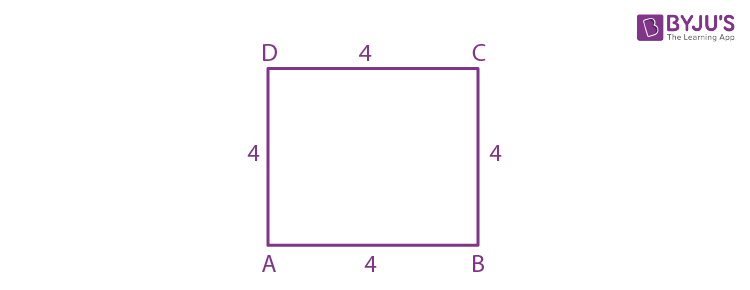Let z = x+iy

CA2 = AB2+BC2

22x2+22y2 = 32

x2+y2 = 8

√(x2+y2 ) = 2√2

17.If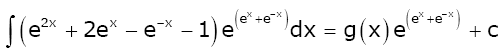where c is a constant of integration, then g(0) is equal to :

a) 2
b) e
c) 1
d) e2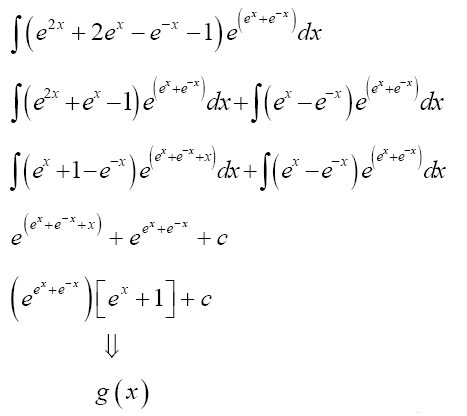⇒ g(0) = 2

18. The negation of the Boolean expression x ↔ y is equivalent to:

a) (x˄y) ˄(∼x˅∼y)
b) (x˄y) ˅ (∼x˄∼y)
c) (x˄∼y) ˅ (∼x˄∼y)
d) (∼x˄y) ˅ (∼x˄∼y)

As we know

p ↔ q = (p→ q) ˄ (q → p)

∼(p ↔ q) = (p˄∼q) ˅ (∼p˄q)

⇒ so, ∼(x ↔ ∼y) = (x˄y) ˅ (∼x˄∼y)

19. If α is positive root of the equation, p(x) = x2-x-2 = 0, then

$$\begin{array}{l}\lim_{x\to\alpha ^{+} }\frac{\sqrt{(1-\cos p(x))}}{x+\alpha -4}\end{array}$$
is equal to:

a) 1/2
b) 3/√2
c) 3/2
d) 1/√2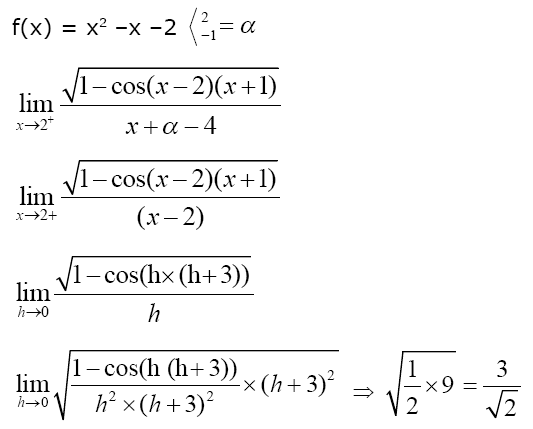20. If the co-ordinates of two points A and B are (√7, 0) and (-√7, 0) respectively and P is any point on the conic, 9x2+16y2 = 144, then PA+PB is equal to :

a) 6
b) 16
c) 9
d) 8

(x2/16)+(y2/9) = 1

e = √(1-9/16)

= √7/4

F1(√7, 0), F2(-√7, 0)

PF1+PF2 = 2a

PA+PB = 2×4 = 8

21. The natural number m, for which the coefficient of x in the binomial expansion of (xm+1/x2)22 is 1540 is..

Tr+1 = 22Cr (xm)22-r(1/x2)r

= 22Cr (x)22m-mr-2r

Given 22Cr = 1540 = 22C19

⇒ r = 19

Since 22m-rm-2r = 1

⇒ m = (2r+1)/(22-r)

m = 13 (At r = 19)

22. Four fair dice are thrown independently 27 times. Then the expected number of times, at least two dice show up a three or a five, is

P(at 2, 3 or 4) = 4C2 (2/6)2(4/6)2+ 4C3 (2/6)3(4/6)1+ 4C4 (2/6)4

= 6×(1/9)×(4/9)+4×(1/27)×(2/3)+(1/81)

= 33/81

= 11/27

⇒ nP ⇒11

23. Let f(x) = x[x/2], for -10<x<10, where [t] denotes the greatest integer function. Then the number of points of discontinuity of f is equal to

f(x) = x[x/2]

x∈ (-10, 10)

x/2 ∈(-5,5) → 9 integers

Check continuity at x = 0

f(0) = 0

f(0+) = 0

f(0) = 0

continuous at x = 0.

Function will be discontinuous when

x/2 =

$$\begin{array}{l}\pm 4, \pm 3, \pm 2, \pm 1\end{array}$$

-4, -3, -2, -1, 0, 1, 2, 3, 4

8 points of discontinuity

24. The number of words, with or without meaning, that can be formed by taking 4 letters at a time from the letters of the word ’SYLLABUS’ such that two letters are distinct and two letters are alike, is

SS, Y, LL, A, B, U

ABCC type words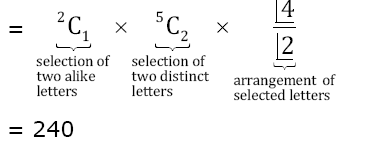25. If the line, 2x-y+3 = 0 is at a distance 1/√5 and 2/√5 from the lines 4x-2y+α = 0 and 6x-3y+β = 0, respectively, then the sum of all possible values of α and β is

L1 : 2x-y+3 = 0

L2 : 4x-2y+α = 0

L3 : 6x-3y+β = 0

|(α/2)-3|/√5 = 1/√5

⇒ (α/2)-3 = 1, -1

⇒ α = 8, 4

|(β/3)-3|/√5 = 2/√5

⇒ (β/3)-3 = 2, -2

⇒ β = 15, 3

sum of all possible values of α and β = 8+4+15+3 = 30